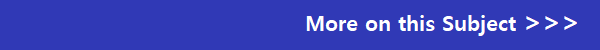## AQA GCSE Maths Past Papers

AQA GCSE Maths (9-1)  (8300) past exam papers. If you are not sure what tier you are sitting foundation or higher check with your teacher. You can download the AQA maths GCSE past papers and marking schemes by clicking on the links below.

AQA GCSE Mathematics (8300) June 2022

Paper 1: Non-Calculator  8300/1F - Foundation

Paper 1: Non-Calculator  8300/1H - Higher

Paper 2: Calculator  8300/2F - Foundation

Paper 2: Calculator  8300/2H - Higher

Paper 3: Calculator  8300/3F - Foundation

Paper 3: Calculator  8300/3H - Higher

AQA GCSE Mathematics (8300) November 2021 (these papers are labelled as June 2021)

Paper 1: Non-Calculator  8300/1F - Foundation

Paper 1: Non-Calculator  8300/1H - Higher

Paper 2: Calculator  8300/2F - Foundation

Paper 2: Calculator  8300/2H - Higher

Paper 3: Calculator  8300/3F - Foundation

Paper 3: Calculator  8300/3H - Higher

AQA GCSE Mathematics (8300) November 2020 (these papers are labelled as June 2020)

Paper 1: Non-Calculator  8300/1F - Foundation

Paper 1: Non-Calculator  8300/1H - Higher

Paper 2: Calculator  8300/2F - Foundation

Paper 2: Calculator  8300/2H - Higher

Paper 3: Calculator  8300/3F - Foundation

Paper 3: Calculator  8300/3H - Higher

AQA GCSE Mathematics (8300) June 2019

Paper 1: Non-Calculator  8300/1F - Foundation

Paper 1: Non-Calculator  8300/1H - Higher

Paper 2: Calculator  8300/2F - Foundation

Paper 2: Calculator  8300/2H - Higher

Paper 3: Calculator  8300/3F - Foundation

Paper 3: Calculator  8300/3H - Higher

AQA GCSE Mathematics (8300) November 2018

Paper 1: Non-Calculator  8300/1F - Foundation

Paper 1: Non-Calculator  8300/1H - Higher

Paper 2: Calculator  8300/2F - Foundation

Paper 2: Calculator  8300/2H - Higher

Paper 3: Calculator  8300/3F - Foundation

Paper 3: Calculator  8300/3H - Higher

AQA GCSE Mathematics (8300) June 2018

Paper 1: Non-Calculator  8300/1F - Foundation

Paper 1: Non-Calculator  8300/1H - Higher

Paper 2: Calculator  8300/2F - Foundation

Paper 2: Calculator  8300/2H - Higher

Paper 3: Calculator  8300/3F - Foundation

Paper 3: Calculator  8300/3H - Higher

AQA GCSE Mathematics (8300) November 2017

Paper 1: Non-Calculator  8300/1F - Foundation

Paper 1: Non-Calculator  8300/1H - Higher

Paper 2: Calculator  8300/2F - Foundation

Paper 2: Calculator  8300/2H - Higher

Paper 3: Calculator  8300/3F - Foundation

Paper 3: Calculator  8300/3H - Higher

AQA GCSE Mathematics (8300) June 2017

Paper 1: Non-Calculator  8300/1F - Foundation

Paper 1: Non-Calculator  8300/1H - Higher

Paper 2: Calculator  8300/2F - Foundation

Paper 2: Calculator  8300/2H - Higher

Paper 3: Calculator  8300/3F - Foundation

Paper 3: Calculator  8300/3H - HigherAQA GCSE Mathematics (8300) Specimen Papers

Paper 1: Non-Calculator  8300/1F - Foundation

Paper 1: Non-Calculator  8300/1H - Higher

Paper 2: Calculator  8300/2F - Foundation

Paper 2: Calculator  8300/2H - Higher

Paper 3: Calculator  8300/3F - Foundation